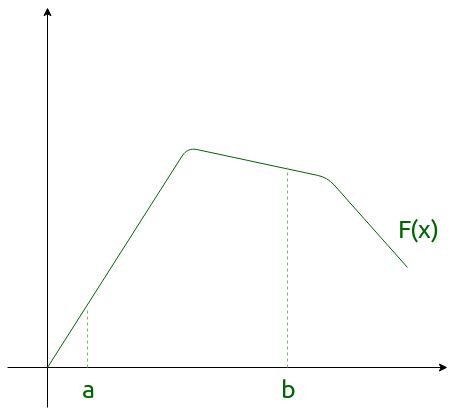Open in App
Not now

# Definite Integral | Mathematics

• Last Updated : 29 Nov, 2022

Definite integrals are the extension after indefinite integrals, definite integrals have limits [a, b]. It gives the area of a curve bounded between given limits., It denotes the area of curve F(x) bounded between a and b, where a is the lower limit and b is the upper limit.Note: If f is a continuous function defined on the closed interval [a, b] and F be an anti derivative of f.

ThenHere, the function f needs to be well defined and continuous in [a, b].

Example:Solution:1.2.3.4.5.6.7.8.9.These properties can be used directly to find the value of a particular definite integral and also interchange to other forms if required.

My Personal Notes arrow_drop_up